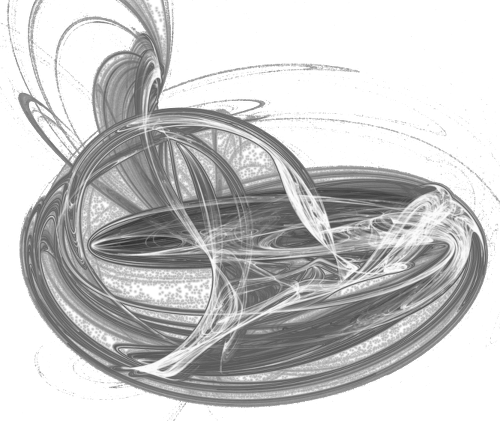Clojure has a really nice set of features for selectively using parts of an object system. It's nice not to have to buy into full OOP, and use only what's needed. The Clojure Cookbook, by Luke VanderHart and Ryan Neufeld covers some of these very nicely, and we'll explore some of those in this post with an eye towards an implementation in LFE.

In particular, we'll be looking at section 3.9 of the Cookbook, "Building Functions with Polymorphic Behaviour". Our discussion in this post will be limited to:

• map-based dispatch, and
• multi-methods

The Clojure example given in the Cookbook is also one used as an example in Joe Armstrong's book Programming Erlang (2nd Edition): calculating the area of shapes.

## Map-based dispatch

Here's the Clojure example which uses maps to not only provide the defining characteristics of a given shape, but to indicate the type of shape and thus decide which code to execute:

``````(defn area
"Calculate the area of a shape"
[shape]
(condp = (:type shape)
:triangle (* (:base shape) (:height shape) (/ 1 2))
:rectangle (* (:length shape) (:width shape))))``````

Usage is as follows:

``````user=> (area {:type :triangle :base 2 :height 4})
4N
user=> (area {:type :rectangle :length 2 :width 4})
8N``````

This is easily translated to LFE:

``````(defun area
"Calculate the area of a shape"
((`#m(type triangle base ,b height ,h))
(* b h (/ 1 2)))
((`#m(type rectangle length ,l width ,w))
(* l w)))``````

We're using the new maps data structure, but could just as easily have used tuples.

For a little bit more dispatch:

``````(defun area
"Calculate the area of a shape"
((`#m(type triangle base ,b height ,h))
(area-triangle b h))
((`#m(type rectangle length ,l width ,w))
(area-rectangle l w)))

(defun area-triangle (b h)
(* b h (/ 1 2)))

(defun area-rectangle (l w)
(* l w))``````

These are both used very similarly as the Clojure example:

``````> (area #m(type triangle base 2 height 4))
4.0
> (area #m(type rectangle length 2 width 4))
8``````

The critique the Cookbook authors have for this is what you might expect: dispatch and implementation are mixed, providing a "pattern" that is mostly unsustainable in large codebases which indicate the use of polymorphic functions.

The practical upshot of this means that we have two places code needs to be touched any time support for a new shape is added: we need to create the function which calculates the area, and we need to tell the dispatch function about it.

## Multi-methods

Clojure overcomes this difficulty with multi-methods: define an abstraction with `defmulti` and then define an implementation with `defmethod`:

``````(defmulti area
"Calculate the area of a shape"
:type)

(defmethod area :rectangle [shape]
(* (:length shape) (:width shape)))

(defmethod area :circle [shape]

Usage is the same as the last Clojure example:

``````user=> (area {:type :rectangle :length 2 :width 4})
8
user=> (area {:type :circle :radius 2})
12.566370614359172``````

Neither Erlang nor LFE have this type of functionality built in. However, this is just the sort of problem the los project was intended to address. A new issue was opened to add support for `defmulti`/`defmethod`, with some non-macro examples of how to accomplish this. We'll outline this below, but use maps instead of tuples.

First, we define a general dispatch function which can handle not only any type of shape, but any future function we may want our shapes to support:

``````(defun dispatch (fname type args)
(call (MODULE) (list_to_atom (++ (atom_to_list fname)
"-"
(atom_to_list type))) args))``````

Notice the call to `(MODULE)` – this approach requires saving this code to a module and compiling it (in other words, a simple copy/paste in the REPL won't work for this example).

Next, we'll add an abstract area function:

``````(defun area
(((= `#m(type ,type) args))
(dispatch 'area type (maps:remove 'type args))))``````

With this done, let's implement our area functions for the two shapes we've seen so far:

``````(defun area-triangle
((`#m(base ,b height ,h))
(* b h (/ 1 2))))

(defun area-rectangle
((`#m(length ,l width ,w))
(* l w)))``````

This code has actually been provided in the `examples` directory of the los project. To run in in the REPL, just do the following (assuming you have Erlang, `rebar`, and `lfetool` installed, or course …). To run the following, you will need the latest version of lfetool:

``````\$ git clone https://github.com/lfex/los.git
\$ cd los
\$ make repl``````

That will get the deps, compile everything, and then dump you into the REPL, at which point we can compile and slurp the example:

``````> (c "examples/no-macros/polymorph.lfe")
#(ok polymorph)
> (slurp "examples/no-macros/polymorph.lfe")
#(ok polymorph)``````

Now we're ready to try it out:

``````> (area #m(type triangle base 2 height 4))
4.0
> (area #m(type rectangle length 2 width 4))
8``````

There are also two other functions supplied in that module:

``````(defun area-square
((`#m(side ,s))
(* s s)))

(defun area-circle
(* (math:pi) r r)))``````

No changes had to be made to either `area` or `dispatch` for them. Execution is as one might expect:

``````> (area #m(type square side 2))
4
> (area #m(type circle radius 2))
12.566370614359172``````

Furthermore, we can add new functions without changing the `dispatch` function:

``````(defun perim
(((= `#m(type ,type) args))
(dispatch 'perim type (maps:remove 'type args))))

(defun perim-rectangle
((`#m(length ,l width ,w))
(* 2 (+ l w))))

(defun perim-circle
(* 2 (math:pi) r)))``````

Let's try these out:

``````> (perim #m(type rectangle length 4 width 2))
12
> (perim #m(type circle radius 1))
6.283185307179586``````

## Next Steps

Two things need to be done to convert this to useful functionality in the los library:

1. Create macros which will generate a generic function (via `defmulti`) and concrete implementation (via `defmethod`).
2. Tweak the examples so that any number of "type" args can be passed.

11 July 2015

design